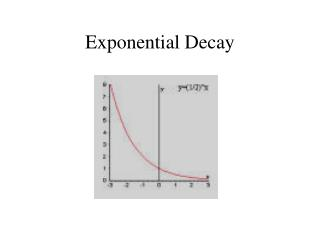DownloadDownload PresentationExponential Decay

# Exponential Decay

Télécharger la présentation## Exponential Decay

- - - - - - - - - - - - - - - - - - - - - - - - - - - E N D - - - - - - - - - - - - - - - - - - - - - - - - - - -
##### Presentation Transcript

1. Exponential Decay

2. Exponential Decay A quantity is decreasing exponentially if it decreases by the same percent r in each unit of time t Decay factor Modeled by the function: y = C(1 – r)t time C –initial amount before decay occurs Initial value Decay rate (decimal) r – decay rate (as a decimal) t– represents time (1 – r) – represents the decay factor

3. Example 1 : Write an Exponential Decay Model You bought a car for \$16,000. You expect the car to lose value, or depreciate, at a rate of 12% per year. Write an exponential model representing the situation. Solution: t = the years of ownership C = initial value \$16,000 r = decay rate 12% or 0.012 y = C(1 – r) t Write exponential decay model = 16000(1 – 0.12) t Substitute 16,000 for C and 0.12 for r. = 16,000(0.88)t

4. Lets do your own exponential decay model Let’s say you bought a used car for \$5,000. The car depreciates at the rate of 15% per year. Write an exponential model to represent the car’s value.

5. Use the exponential model created in Example 1 to find the value of the car after 8 years. y = 16,000(0.88)t This is the current model To find the value after 8 years substitute 8 for t. = 16,000(0.88)8 Substituted 8 for t…now use a calculator to find answer  \$5,754 This is all your car will be worth after 8 years!!!

6. Graphs of Exponential Decay Models • Graph the model in example 1. • Use the graph to estimate the value of your car after 5 years. Solution: Make a table of values as before 0 2 4 6 8 16,000 x 16,000 14,000 y 12,390 9595 7430 5754 Value (dollars) 12,000 Now lets estimate the value after 5 years. 10,000 8000 Start at 5 on the years axis (x) 6000 4000 Then over to y -axis 0 2 4 6 8 10 At 5 years, a little over \$8,000. time (years)

7. Now you try to graph the exponential decay model from the car dealer ship problem!

8. Example 2 You own a business that earned \$105,000 in 2003. The earnings of your business begin to decrease by 2% for the next 10 years. Write an exponential decay model to represent the decreasing annual earnings of your business. Then evaluate how much your business would make in 2013 (10 years later). y = C(1 – r) t Write exponential decay model = 105,000(1 – 0.02) 10 Substitute 105,000 for C, 0.02 for r, and 10 for t. = 105,000(.98) 10 Subtract 1 – 0.02 = 85,792.64 Solve Your business only made \$85,792.64 ten years later!! What influences may have caused this loss?